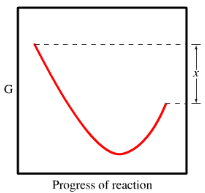# Problem: The diagram for the free energy of the reactionA(g) + B(g) ⇌ AB(g)The reaction progress starts on the left with pure reactants, A and B, each at 1 atm and moves to pure product, AB, also at 1 atm on the right. Select the true statements.a. The difference between the top left of the curve and the minimum of the curve corresponds to ΔG standard.b. The "x" on the graph corresponds to ΔG standard.c. The minimum on the graph corresponds to the equilibrium position of the reaction.d. The entropy change for the reaction is positive.e. At equilibrium, all of A and B have reacted to form pure AB.

###### FREE Expert Solution
91% (380 ratings)
###### FREE Expert Solution
91% (380 ratings)###### Problem Details

The diagram for the free energy of the reaction

A(g) + B(g) ⇌ AB(g)

The reaction progress starts on the left with pure reactants, A and B, each at 1 atm and moves to pure product, AB, also at 1 atm on the right.

Select the true statements.

a. The difference between the top left of the curve and the minimum of the curve corresponds to ΔG standard.
b. The "x" on the graph corresponds to ΔG standard.
c. The minimum on the graph corresponds to the equilibrium position of the reaction.
d. The entropy change for the reaction is positive.
e. At equilibrium, all of A and B have reacted to form pure AB.What scientific concept do you need to know in order to solve this problem?

Our tutors have indicated that to solve this problem you will need to apply the Gibbs Free Energy concept. You can view video lessons to learn Gibbs Free Energy. Or if you need more Gibbs Free Energy practice, you can also practice Gibbs Free Energy practice problems.

What is the difficulty of this problem?

Our tutors rated the difficulty ofThe diagram for the free energy of the reactionA(g) + B(g) ⇌...as medium difficulty.

How long does this problem take to solve?

Our expert Chemistry tutor, Dasha took 6 minutes and 4 seconds to solve this problem. You can follow their steps in the video explanation above.

What professor is this problem relevant for?

Based on our data, we think this problem is relevant for Professor Du's class at UGA.